Homework Help Question & Answers

1. Suppose Yi, ½ . . . , Yn is a random sample of n independent observations from a distribution ...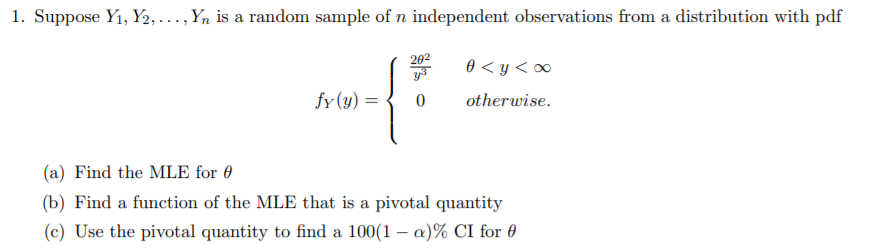1. Suppose Yi, ½ . . . , Yn is a random sample of n independent observations from a distribution with pdf 202 fY()otherwise. (a) Find the MLE for θ (c) Use the pivotal quantity to find a 100(1-a)% CI for θ

Solution: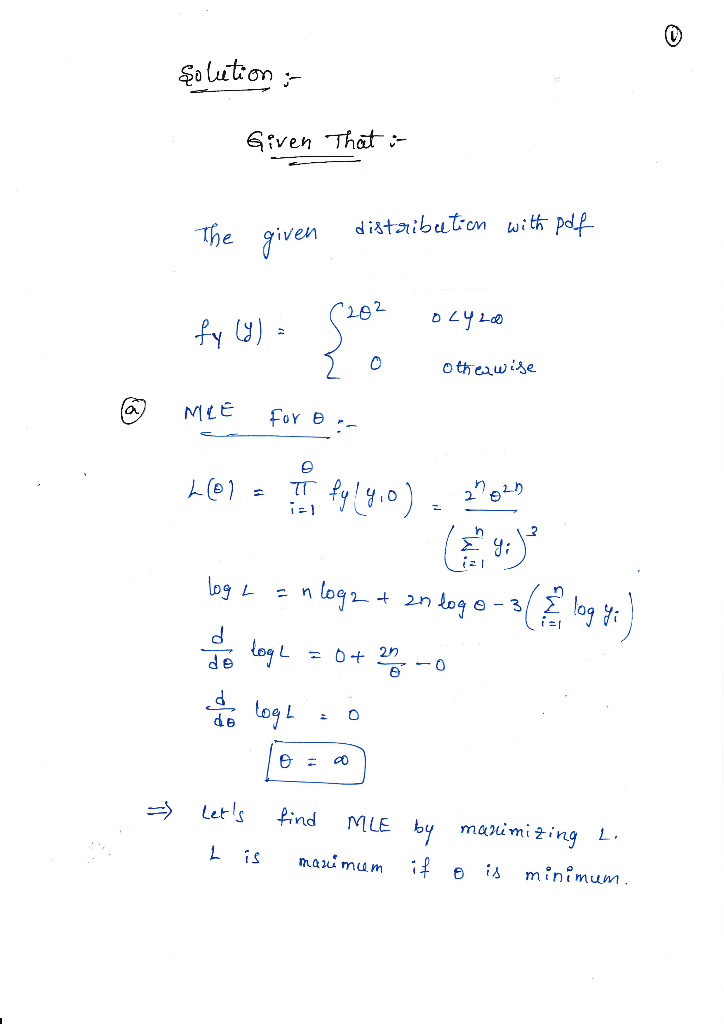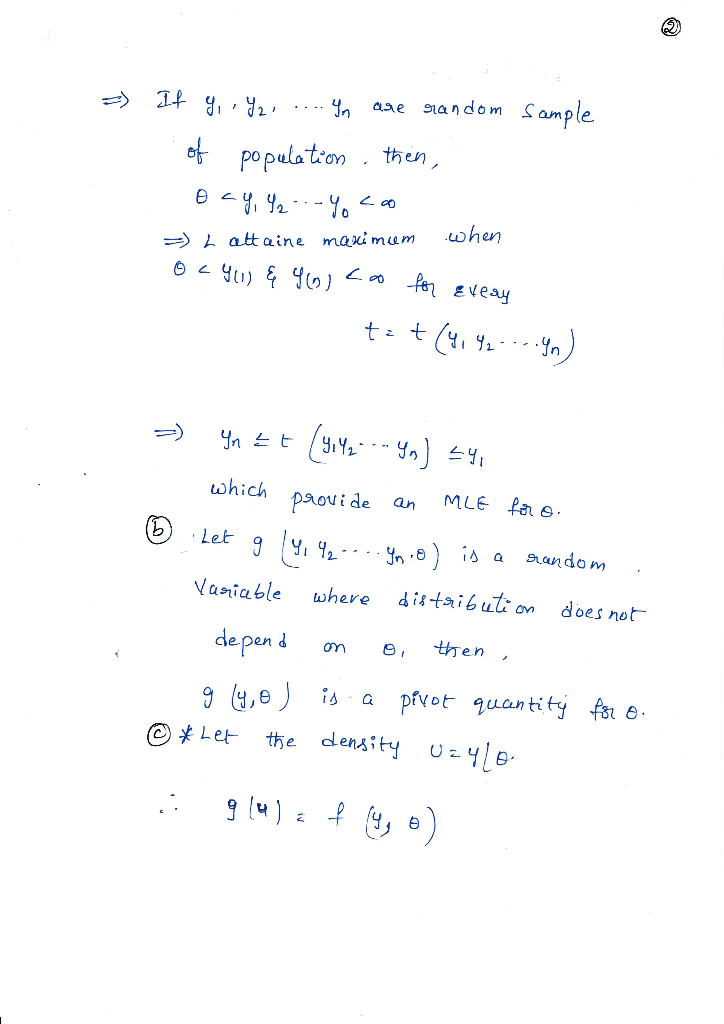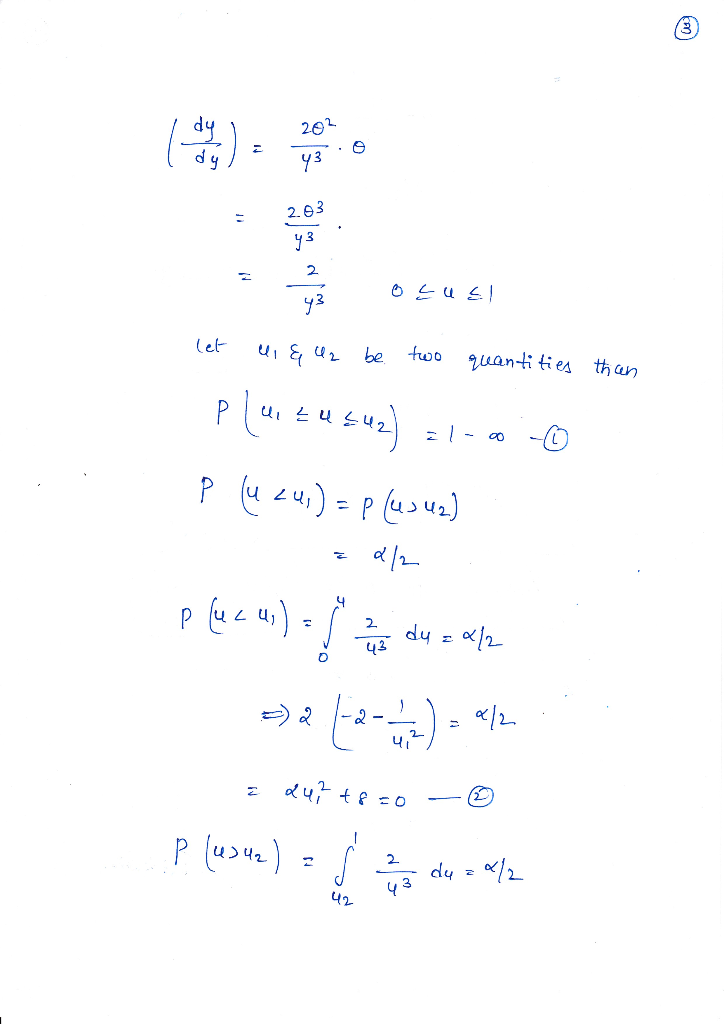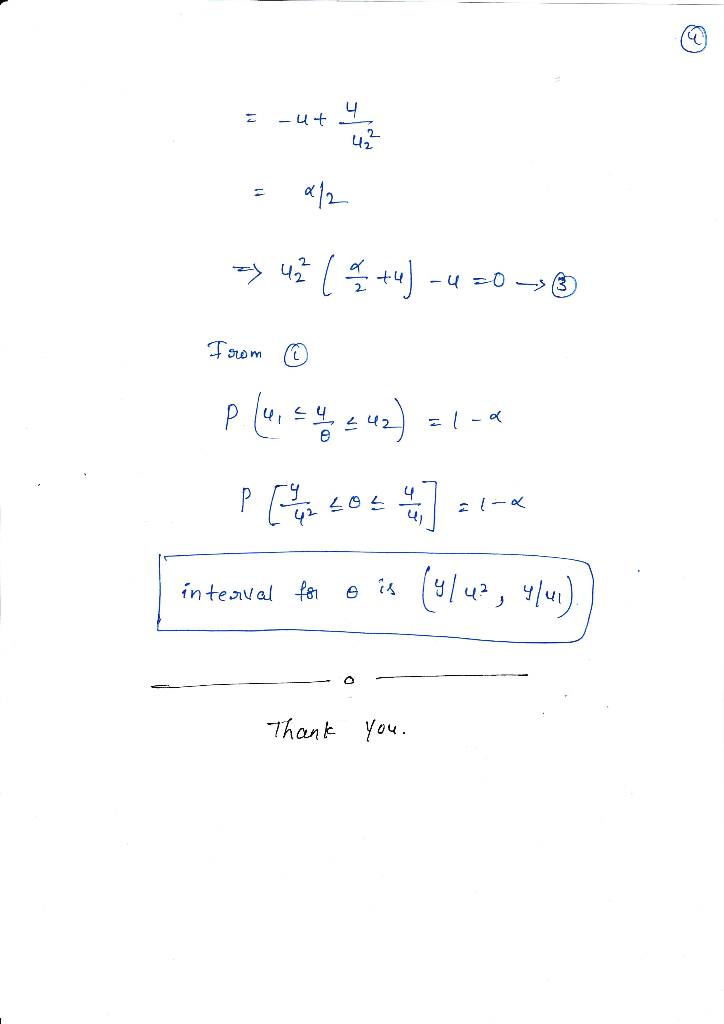Add Answer of: 1. Suppose Yi, ½ . . . , Yn is a random sample of n independent observations from a distribution ...
More Homework Help Questions Additional questions in this topic.

• Suppose you take a random sample of 30 individuals from a large population. For this sample, the sample mean is 4.2 and sample variance is 49. You wish to estimate the unknown population mean µ. (a) C...

Need Online Homework Help?

Get FREE EXPERT Answers
WITHIN MINUTES
Related Questions